## The Wythoff Symbol

All but one of the uniform polyhedra can be described by a Wythoff symbol. The Wythoff symbol contains three rational numbers p, q, and r, all larger than 1. If two of these numbers are equal to 2, the third one is arbitrary, otherwise only the numerators 2, 3, 4, and 5 can occur, and 4 and 5 cannot occur together. There are only finitely many ways to choose such p, q, and r. There are four kinds of Wythoff symbols (plus one exceptional case), described below, written

If the numbers p, q, and r in the Wythoff symbol are integers, the corresponding polyhedron is convex. All convex uniform polyhedra have been known since antiquity.

### Case 1: p|q r: Regular and Quasi-Regular Polyhedra

The regular and quasi-regular polyhedra have vertex configuration {q, r, q, r, ..., q, r} (with 2n terms, where p=n/d).That is, they consist of two kinds of faces, arranged alternatingly. If q=r, they are regular.

#### Examples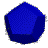The dodecahedron has the formula 3|2 5, therefore, the vertex configuration is {2, 5, 2, 5, 2, 5}. After removing trivial faces, simply {5, 5, 5} remains, that is, 3 pentagons.The cuboctahedron has the formula 2|3 4, therefore, the vertex configuration is {3, 4, 3, 4}.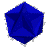The great dodecahedron has the formula 5/2|2 5, therefore, the vertex configuration is {5, 5, 5, 5, 5}/2, that is, five pentagons, but each vertex is traversed twice (in the form of a pentagram). It is regular.

### Case 2: p q|r: Semiregular Polyhedra

The semiregular polyhedra have vertex configuration {p, 2r, q, 2r}.

#### ExamplesThe truncated tetrahedron has the formula 2 3|3, therefore, the vertex configuration is {2, 6, 3, 6}. After removing trivial faces, simply {6, 3, 6} remains, that is, two hexagons and one triangle.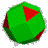The rhombicuboctahedron has the formula 3 4|2, therefore, the vertex configuration is {3, 4, 4, 4}.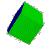The prisms are also in this class; they have the formula 2 n|2. They have vertex configuration {2, 4, n, 4}, or {4, 4, n} after removing trivial faces. Here, n=5.

### Case 3: p q r|: Even-Faced Polyhedra

The even-faced polyhedra have vertex configuration {2p, 2q, 2r}.

#### Examples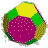The truncated cuboctahedron has the formula 2 3 4|, therefore, the vertex configuration is {4, 6, 8}.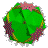The great truncated cuboctahedron has formula 4/3 2 3|, therefore, the vertex configuration {8/3, 4, 6}, that is, a square, hexagon, and an eight-pointed star.

### Case 4: |p q r: Snub Polyhedra

The snub polyhedra have vertex configuration {3, p, 3, q, 3, r}. They contain three connecting triangles. Many of them don't have mirror symmetry, that, is there is a left and right form.

#### Examples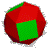The snub cube has the formula |2 3 4, therefore, the vertex configuration is {2, 3, 3, 3, 4, 3}. After removing trivial faces, {3, 3, 3, 3, 4} remains, that is, four triangles and one square.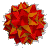The great snub dodecicosidodecahedron has the formula |5/3 5/2 3, therefore, the vertex configuration is {5/3, 3, 5/2, 3, 3, 3}, that is, two pentagrams (one of them retrograde) and four triangles.

### The Exception: |3/2 5/3 3 5/2: the Great DirhombicosidodecahedronThere is one uniform polyhedron that cannot be constructed with Wythoff's method. It is the only one with more than six faces around a vertex (it has 8 of them!). It has vertex configuration {4, 5/3, 4, 3, 4, 5/2, 4, 3/2}. It is denoted by the pseudo Wythoff symbol (|3/2 5/3 3 5/2) and named the Great Dirhombicosidodecahedron. It can be found by a modification of (|5/3 5/2 3). The Great Dirhombicosidodecahedron consists of 40 triangles (20 of them retrograde), 60 hemispherical snub squares, and 24 pentagrams (12 of them retrograde). It also has the largest number of faces (124) and edges (240) of all uniform polyhedra. The squares come in 20 coplanar pairs, that is, two squares each lie in the same plane, rotated against each other by 38.17 degrees.Programs and high-resolution images for uniform polyhedra are available in the book The Mathematica Programmer II by R. Maeder.All 75 uniform polyhedra, with background information, a clickable map, and animations.

A service provided by MathConsult AG, http://www.mathconsult.ch/.